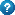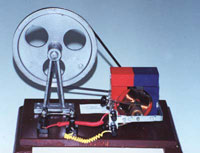The function of the generators is based on the principle of induction. Coils rotate within magnetic field. The rotation is achieved through various means such as wind (wind-turbines), water (hydroelectric plants) or steam (thermo-electric, nuclear, geothermal plants). The mechanical energy is transformed first into kinetic to the motor and the coils
and then into electrical.Almost all generators used to generate electric current, produce alternating current (which usually has a frequency of 50 or 60 cycles per second).

Initially, the coil is still and the generated electric current is zero. As the coil rotates the electric current produced increases gradually, reaching a maximum positive value, then begins to decrease zero (when the coil is "upside down in the magnetic field), then reaches a maximum negative value returns to zero and the "cycle" is repeated. This circle is repeated sometimes the second. If the "weapons", i.e. the coils, consisting of 2 wires together that form right angles, and connected to different external links, then 2 currents are produced where the one has its maximum value when the other is zero. This is called an alternating electrical current with two phases.

If we have 3 coils connected by angle of 120 degrees, then the generated electric current has 3 phases, the famous three-phase alternating electric current.
Theoretically we can produce electric current with more phases, but the three-phase that is what usually produced in today's factories.

The function of the generator is based on the principle of induction discovered in 1831 by the English scientist Michael Faraday.© 2003, Despina M. Garyfallidou Athens-Greece